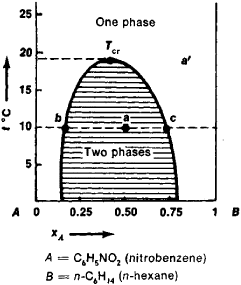# Solubility Diagram

The following article is from The Great Soviet Encyclopedia (1979). It might be outdated or ideologically biased.

## Solubility Diagram

a graphic representation of the equilibrium composition of solutions as a function of temperature, pressure, or other parameter characterizing the external conditions.

The solubility diagram is a special type of phase diagram, the latter being widely used in chemical thermodynamics. A solubility diagram for a system made up of two partially miscible liquid components A and B, nitrobenzene and n-hexane, is shown in Figure 1. Each point a’ outside the shaded region corresponds to an unsaturated solution of one component in the other; each point on the curve bounding this region represents a saturated solution; and each point inside the shaded region corresponds to a two-phase system from the layer of the saturated solution of A in B, whose composition is denoted by point b, and from the layer of the saturated solution of B in A, whose composition is denoted by point c.Figure 1

Complete miscibility occurs in many systems at temperatures above a certain TCT —the critical solution temperature. The compositions of both layers are identical at this temperature. If the curve bounding the two-phase region has a maximum, TCT is called the upper critical solution temperature (Figure 1); if it has a minimum, it is called the lower critical solution temperature. The solubility diagrams for certain systems, for example, water-nicotine, contain both critical temperatures.

Mentioned in ?
References in periodicals archive ?
The solubility of fenofibrate in every HP[beta]CD solution was calculated and a phase solubility diagram was drawn between solubility of fenofibrate and different concentrations of HP[beta]CD.
The apparent stability constants, [K.sub.1:1], obtained from the slope of the linear phase solubility diagram was 630.0006 [M.sup.-1] for HP[beta]CD [Eq.
According to the model presented by Higuchi et al the phase solubility diagram of QpE belongs to AL type suggesting that the inclusion complex was formed with a molar ratio of 1:1.
The apparent stability constant, Ks, was calculated from the phase solubility diagram with the assumption of 1: 1 stoichiometry, according to (1):
As can be seen, it shows AL-type solubility diagram as the pyrimethanil solubility increases with increasing HP-[beta]-CD concentrations, according to the classification established by Higuchi and Connors .
SAP solubility diagram. In this plot, data points more tightly cluster around the solubility (or observed constant IAP in the case of AlOHS[O.sub.4]) lines, as the plot accounts for more variables than the Al activity v.
Solubility diagrams were constructed using the equations for amorphous aluminium hydroxide, alunite, and AlOHS[O.sub.4] plus equations and p[K.sup.0] values from the literature for alunogen (Nordstrom 1982) and basaluminite (Adams and Rawajfih 1977).
The stoichiometry of pesticide-cyclodextrin complexes and their stability or binding constants are frequently obtained from the phase solubility diagrams. These phase solubility studies were performed by the method reported by Higuchi and Connors .
The phase solubility diagrams were obtained plotting the equilibrium concentrations of terbuthylazine against the concentration of the respective CD.
The apparent stability constants, [K.sub.s], were calculated from phase solubility diagrams with the assumption of 1:1 stoichiometry, according to the following equation:

Site: Follow: Share:
Open / Close